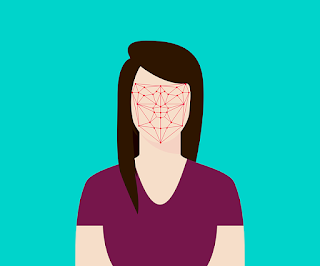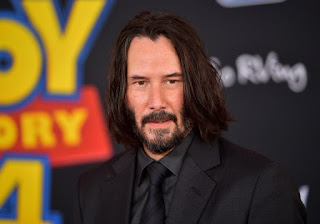### Snapchat/Instagram type filters using Deep Learning

Have you used filters on Snapchat and Instagram? Have you ever wondered how do those filters work? Have you ever wondered how Instagram or Snapchat detect our faces and impose dog filters, masks, etc on our face?

In this post, we will design such type of filter which will detect the face than impose a Santa beard and the swag glasses on the face.### Required things and datasets

To do this project we require some knowledge of python and its packages (like Numpy, Pandas, cv2) and some deep learning (and packages like Tensorflow and Keras) knowledge. You can find the dataset here.

### How we will do this? (Process)

First of all, we will train our model to detect the key features on the face like lips, eyes, eyebrows, and nose. When our model is trained enough to detect these key feature we can use these features to impose beards and glasses on the face. The whole process is:

• Detect the face (we will be using Haar Face Detector)
• Detect key features (like eyes, lips) on the face
• Use these key feature to impose the filter

### Coding

#### Create and train model

Train the model to detect the key features from a face and remove all the null value rows from training dataset

train_nonull = training[training.isna().sum(axis=1) < 1]
print("Non null rows: ", len(train_nonull))

We got about 2140 rows which have no null values. Then we prepare the training data and test data for training the model.

# Training Data
train_images = []
train_points = []

for i in range(len(train_nonull)):
point = train_nonull.iloc[i, :-1]
point = point/96 - 0.5
train_points.append(point)
img = np.array(train_nonull.iloc[i, -1].split(' '), dtype=np.int)
img = img.reshape(96,96)/255
train_images.append(img)

train_images = np.array(train_images)
train_points = np.array(train_points)

# Test data
imgs_test = []
for i in range(len(test)):
test_image = test.iloc[i,-1]
test_image = np.array(test_image.split(' ')).astype(int)
test_image = np.reshape(test_image, (96,96))
test_image = test_image/255
imgs_test.append(test_image)

imgs_test = np.array(imgs_test)We can see the images and “red X” mark the key features. Now that we have the data. We can also add the mirrored images of these as we have very fewer data.

def augment(img, points):
f_img = img[:, ::-1]
pts = points.copy()
for i in range(0,len(pts),2):
x_renorm = (points[i]+0.5)*96
dx = x_renorm - 48
x_renorm_flipped = x_renorm - 2*dx
pts[i] = x_renorm_flipped/96 - 0.5
return f_img, pts

aug_imgs_train = []
aug_points_train = []

for i in range(len(train_images)):
f_img, f_points = augment(train_images[i], train_points[i])
aug_imgs_train.append(f_img)
aug_points_train.append(f_points)

aug_imgs_train = np.array(aug_imgs_train)
aug_points_train = np.array(aug_points_train)

imgs_total = np.concatenate((train_images, aug_imgs_train), axis=0)
imgs_total = imgs_total.reshape([-1, 96, 96, 1])
points_total = np.concatenate((train_points, aug_points_train), axis=0)

Now that we have the data lets create and train our model. We have used Keras to create our model.

def get_model():
model = tf.keras.Sequential()

model.summary()
return model

get_model function will create our model. Now let’s compile and train our data.

model = get_model()
checkpoint = tf.keras.callbacks.ModelCheckpoint(filepath='weights/checkpoint-{epoch:02d}.hdf5')
model.fit(imgs_total, points_total, epochs=300, batch_size=100, callbacks=[checkpoint])

After 300 epochs, the final result we got was loss: 0.0166, acc: 0.7217
Now let’s check the model on our test images.

We can see that our model is working fine.

#### Now use the model on a real-time image

We have used this image and added filters on this imagegray = cv2.cvtColor(img, cv2.COLOR_BGR2GRAY)

So we read the image and got the faces out of it using haar algo. Now detect the key features and impose the filter on it.

for (x,y,w,h) in faces:
roi_gray = gray[y:y+h, x:x+w]
img_copy = np.copy(img)
img_copy_1 = np.copy(img)
roi_color = img_copy_1[y:y+h, x:x+w]

width_original = roi_gray.shape
height_original = roi_gray.shape

img_gray = cv2.resize(roi_gray, (96, 96))
img_gray = img_gray/255

img_model = np.reshape(img_gray, (1,96,96,1))
keypoints = model.predict(img_model)

x_coords = keypoints[0::2]
y_coords = keypoints[1::2]

x_coords_denormalized = (x_coords+0.5)*width_original
y_coords_denormalized = (y_coords+0.5)*height_original

for i in range(len(x_coords)):
cv2.circle(roi_color, (x_coords_denormalized[i], y_coords_denormalized[i]), 2, (255,255,0), -1)

left_lip_coords = (int(x_coords_denormalized), int(y_coords_denormalized))
right_lip_coords = (int(x_coords_denormalized), int(y_coords_denormalized))
top_lip_coords = (int(x_coords_denormalized), int(y_coords_denormalized))
bottom_lip_coords = (int(x_coords_denormalized), int(y_coords_denormalized))
left_eye_coords = (int(x_coords_denormalized), int(y_coords_denormalized))
right_eye_coords = (int(x_coords_denormalized), int(y_coords_denormalized))
brow_coords = (int(x_coords_denormalized), int(y_coords_denormalized))

beard_width = np.abs(right_lip_coords - left_lip_coords)
glasses_width = np.abs(right_eye_coords - left_eye_coords)

img_copy = cv2.cvtColor(img_copy, cv2.COLOR_BGR2BGRA)

# santa
(h_santa, w_santa) = santa_filter.shape[:2]
width_santa = beard_width*3
r_santa = width_santa / float(w_santa)
dim = (width_santa, int(h_santa * r_santa))

santa_filter = cv2.resize(santa_filter, dim)
sw,sh,sc = santa_filter.shape

beard_centre_x = x + (left_lip_coords + right_lip_coords)/2
beard_centre_y = y+ (left_lip_coords + right_lip_coords)/2
beard_up = 0.2 * height_original

sw_changed = sw
if int(beard_centre_y - beard_up)+sw > img_copy.shape:
sw_changed = img_copy.shape - int(beard_centre_y - beard_up)
sh_changed = sh
if int(beard_centre_x - 0.5 * sh) + sh > img_copy.shape:
sh_changed = img_copy.shape - int(beard_centre_x - 0.5 * sh)

for i in range(0,sw_changed):
for j in range(0,sh_changed):
if santa_filter[i,j] != 0:
img_copy[int(beard_centre_y - beard_up) + i, int(beard_centre_x - 0.5 * sh) + j] = santa_filter[i,j]

#glasses
(h_specs, w_specs) = santa_filter.shape[:2]
width_specs = int(glasses_width*1.4)
r_specs= width_specs / float(w_specs)
dim = (width_specs, int(h_specs * r_specs - 0.15*height_original))

specs_filter = cv2.resize(specs_filter, dim)
gw,gh,gc = specs_filter.shape

glass_centre_x = x + (left_eye_coords + right_eye_coords)/2
glass_centre_y = y + (left_eye_coords + right_eye_coords)/2

glass_up = 0.35 * height_original

for i in range(0,gw):
for j in range(0,gh):
if specs_filter[i,j] != 0:
img_copy[int(glass_centre_y - glass_up) + i, int(glass_centre_x - 0.5 * gh) + j] = specs_filter[i,j]

plt.imshow(img_copy)

cv2.imwrite("out.jpg", img_copy)

So let’s check the final output.

So we can see that we applied the filter on this image. So this is how Instagram and Snapchat use filters.
You can check the complete code here (https://github.com/abhimanyu1996/Snapchat-Instagram-type-filters-using-Deep-Learning). Thank you for reading this post.